## Inside the box of every cereal box contains a surprise gift. The store manager assures employees that 22 of the 45 boxes on the shelf have a

Question

Inside the box of every cereal box contains a surprise gift. The store manager assures employees that 22 of the 45 boxes on the shelf have a secret decoder ring and inside the other 23 boxes on the shelf contain a different gift. If two cereal boxes are randomly selected from the shelf to purchase, what is the probability that both of them have the secret decoder ring

in progress 0
2 months 2021-08-19T05:32:25+00:00 1 Answers 0 views 0

0.2333 = 23.33% probability that both of them have the secret decoder ring

Step-by-step explanation:

The cereals are chosen without replacement, which means that the hypergeometric distribution is used to solve this question.

Hypergeometric distribution:

The probability of x successes is given by the following formula: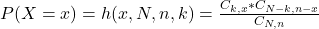In which:

x is the number of successes.

N is the size of the population.

n is the size of the sample.

k is the total number of desired outcomes.

Combinations formula: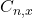is the number of different combinations of x objects from a set of n elements, given by the following formula.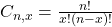In this question:

45 boxes, which means that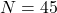22 have the secret decoder ring, which means that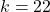Two boxes, which means that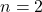What is the probability that both of them have the secret decoder ring?

This is P(X = 2). So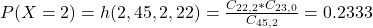0.2333 = 23.33% probability that both of them have the secret decoder ring